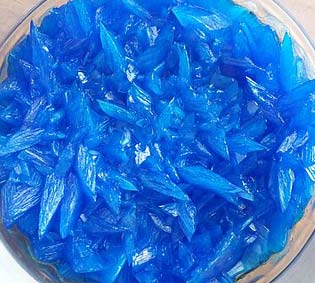Grab a calculator and a copy of the periodic table

(This test has only 12 questions, but may take 15 - 20 mins).

1. An oxide of iron, on reduction, was found to contain 1.12g of iron and 0.32g of oxygen.

Calculate the empirical formula of this iron oxide.
[Relative atomic masses: Fe = 56; O=16]

• A: FeO
• B: Fe2O2
• C: Fe2O
• D: Fe2O3
2. A carbohydrate was found to have an empirical formula CH2O and a relative molecular mass of 120. The molecular formula of the carbohydrate is …
• A.   CH2O
• B.   C2H4O2
• C.   C4H8O4
• D.   C6H12O6

3. An ore of titanium contains 36.8% iron, 31.6% titanium and 31.6% oxygen.

Determine the simplest whole number ratio for the moles of atoms.
[Ar values: Fe = 56; Ti = 48; O = 16]

 Fe Ti O A 1 1 1 B 1 1 2 C 1 1 3 D 4 3 1
4. 1.38g of sodium was burned in oxygen. An oxide of mass 2.34g was formed.

Calculate the moles of sodium and oxygen atoms in the final product.
[Ar of Na = 23; Ar of O = 16]

 Moles of sodium atoms Moles of oxygen atoms A 0.06 0.146 B 0.06 0.06 C 0.102 0.06 D 0.102 0.146

Q5-7:
1.23g of hydrated magnesium sulfate, MgSO4.xH2O, was heated to constant mass in a crucible.
0.60g of anhydrous magnesium sulfate, MgSO4, remained at the end.
[Relative formula masses: MgSO4 = 120; H2O = 18]

5. Calculate the number of moles of anhydrous magnesium sulfate produced.
• A: 0.0050
• B: 0.010
• C: 0.50
• D: 2.0
6. Calculate the number of moles of water released
• A: 0.033
• B: 0.035
• C: 0.068
• D: 0.050
7. Determine the value of ‘x’ in the MgSO4.

Q8+9:

A student produced a sample of copper(II)sulfate crystals from copper(II)oxide and sulfuric acid. She calculated that she should obtain 18.6g of dry crystals but she only actually obtained 12.4g.8. What was her percentage yield?
• A.   1.5%
• B.   150%
• C.   0.67%
• D.   67%
9. Which of the following is NOT a reason for the actual yield of copper(II)sulfate crystals being less than the theoretical yield?
• A.   The reaction between copper(II)oxide and sulfuric acid was incomplete
• B.   Some copper(II)sulfate solution was lost during filtration
• C.   Some copper(II)sulfate solution did not crystallise
• D.   The copper(II)sulfate crystals were still damp
10. A 20g sample of impure calcium carbonate contains 16g of calcium carbonate.

What is the percentage purity of the calcium carbonate?

• A.   4%
• B.   16%
• C.   20%
• D.   80%

Q11-12:
A student used the following reaction to try to produce 20.0g of copper(II)chloride.

CuCO3 + 2HCl  CuCl2 + H2O + CO2

11. What mass of copper(II)carbonate does the student need to react with excess hydrochloric acid to make the 20.0g of copper(II)chloride?

[Mr of CuCl2 = 134.5; Mr of CuCO3 = 123.5]

• A.   18.4g
• B.   20.0g
• C.   24.9g
• D.   36.8g
12. If the percentage yield of copper(II)chloride in this reaction was only 72%, what mass of copper(II)chloride did the student actually produce?
• A.   7.2g
• B.   14.4g
• C.   27.8g
• D.   1440g